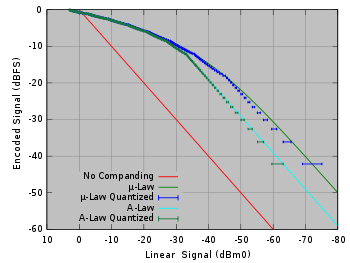# A-law algorithm

﻿
A-law algorithmGraph of μ-law & A-law algorithms

An A-law algorithm is a standard companding algorithm, used in European digital communications systems to optimize, i.e., modify, the dynamic range of an analog signal for digitizing.

It is similar to the μ-law algorithm used in North America and Japan.

For a given input x, the equation for A-law encoding is as follows,$F(x) = \sgn(x) \begin{cases} {A |x| \over 1 + \ln(A)}, & |x| < {1 \over A} \\ \frac{1+ \ln(A |x|)}{1 + \ln(A)}, & {1 \over A} \leq |x| \leq 1, \end{cases}$

where A is the compression parameter. In Europe, A = 87.7; the value 87.6 is also used.

A-law expansion is given by the inverse function,$F^{-1}(y) = \sgn(y) \begin{cases} {|y| (1 + \ln(A)) \over A}, & |y| < {1 \over 1 + \ln(A)} \\ {\exp(|y| (1 + \ln(A)) - 1) \over A}, & {1 \over 1 + \ln(A)} \leq |y| < 1. \end{cases}$

The reason for this encoding is that the wide dynamic range of speech does not lend itself well to efficient linear digital encoding. A-law encoding effectively reduces the dynamic range of the signal, thereby increasing the coding efficiency and resulting in a signal-to-distortion ratio that is superior to that obtained by linear encoding for a given number of bits.

## Comparison to μ-law

The μ-law algorithm provides a slightly larger dynamic range than the A-law at the cost of worse proportional distortion for small signals. By convention, A-law is used for an international connection if at least one country uses it.

Wikimedia Foundation. 2010.

### Look at other dictionaries:

• μ-law algorithm — Companding of μ law and A law algorithms The µ law algorithm (often u law, ulaw, or mu law) is a companding algorithm, primarily used in the digital telecommunication systems of North America and Japan. Companding algorithms reduce the dynamic… …   Wikipedia

• Μ-law algorithm — The µ law algorithm (pronounced myoo law) is a companding algorithm, primarily used in the digital telecommunication systems of North America and Japan. As with other companding algorithms, its purpose is to reduce the dynamic range of an audio… …   Wikipedia

• Law of excluded middle — This article uses forms of logical notation. For a concise description of the symbols used in this notation, see Table of logic symbols. In logic, the law of the excluded middle states that the propositional calculus formula P ∨ ¬ P ( P or not P… …   Wikipedia

• Amdahl's law — Amdahl s law, also known as Amdahl s argument, [Rodgers 85, p.226] is named after computer architect Gene Amdahl, and is used to find the maximum expected improvement to an overall system when only part of the system is improved. It is often used …   Wikipedia

• Constraint algorithm — In mechanics, a constraint algorithm is a method for satisfying constraints for bodies that obey Newton s equations of motion. There are three basic approaches to satisfying such constraints: choosing novel unconstrained coordinates ( internal… …   Wikipedia

• Software patents under United States patent law — Software or computer programs are not explicitly mentioned in United States patent law. In the face of new technologies, decisions of the United States Supreme Court and United States Court of Appeals for the Federal Circuit (CAFC) have sought to …   Wikipedia

• International Data Encryption Algorithm — IDEA An encryption round of IDEA General Designers Xuejia Lai and James Massey …   Wikipedia

• Expectation-maximization algorithm — An expectation maximization (EM) algorithm is used in statistics for finding maximum likelihood estimates of parameters in probabilistic models, where the model depends on unobserved latent variables. EM alternates between performing an… …   Wikipedia

• List of United States patent law cases — This is a list of patent law cases in the United States from 1878 to 2007.Early cases*City of Elizabeth v. American Nicholson Pavement Co. 1878. Prior use does not include experimental use. *Egbert v. Lippmann 1881. Held that public use of an… …   Wikipedia

• Brooks's law — is a principle in software development which says that adding manpower to a late software project makes it later .Frederick P. Brooks, Jr. The Mythical Man Month . 1995. Addison Wesley.] It was coined by Fred Brooks in his 1975 book The Mythical… …   Wikipedia